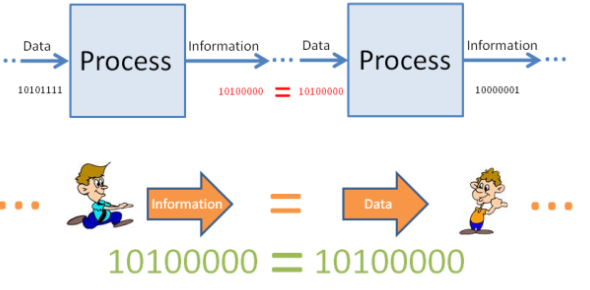# Information Theory And Coding Quiz!

15 Questions | Total Attempts: 133Settings.

• 1.
Tickets numbered 1 to 20 are mixed up and then a ticket is drawn at random. What is the probability that the ticket drawn has a number which is a multiple of 3 or 5?
• A.

1/2

• B.

2/5

• C.

8/15

• D.

9/20

• 2.
A bag contains 2 red, 3 green, and 2 blue balls. Two balls are drawn at random. What is the probability that none of the balls drawn is blue?
• A.

10/21

• B.

11/21

• C.

2/7

• D.

5/7

• 3.
What is the probability of getting a sum of 9 from two throws of a dice?
• A.

1/6

• B.

1/8

• C.

1/9

• D.

1

• 4.
In class, there are 15 boys and 10 girls. Three students are selected at random. The probability that 1 girl and 2 boys are selected, is:
• A.

21/46

• B.

25/117

• C.

1/50

• D.

3/25

• 5.
Susan took two tests. The probability of her passing both tests is 0.6. The probability of her passing the first test is 0.8. What is the probability of her passing the second test given that she has passed the first test?
• A.

0.75

• B.

0.65

• C.

0.80

• D.

0.70

• 6.
In digital communication system, smaller the code rate, _________are the redundant bits.
• A.

Less

• B.

More

• C.

Equal

• D.

Unpredictable

• 7.
If the channel is band-limited to 6 kHz & signal to noise ratio is 16, what would be the capacity of the channel?
• A.

15.15 kbps

• B.

24.74 kbps

• C.

30.12 kbps

• D.

52.18 kbps

• 8.
For a baseband system with transmission rate 'rs' symbols/sec, what would be the required bandwidth?
• A.

Rs / 2 Hz

• B.

Rs / 4 Hz

• C.

Rs / 8 Hz

• D.

Rs / 16 Hz

• 9.
In a recent survey in a Statistics class, it was determined that only 60% of the students attend class on Thursday. From past data, it was noted that 98% of those who went to class on Thursday pass the course, while only 20% of those who did not go to class on Thursday passed the course.
1. What percentage of students is expected to pass the course?
• A.

0.66

• B.

0.24

• C.

0.45

• D.

0.52

• 10.
In a recent survey in a Statistics class, it was determined that only 60% of the students attend class on Thursday. From past data it was noted that 98% of those who went to class on Thursday pass the course, while only 20% of those who did not go to class on Thursday passed the course.
1. Given that a student passes the course, what is the probability that he/she attended classes on Thursday.
• A.

0.61

• B.

0.85

• C.

0.93

• D.

0.55

• 11.
A man is known to speak the truth 2 out of 3 times. He throws a die and reports that the number obtained is a four. Find the probability that the number obtained is actually a four.
• A.

1/6

• B.

3/5

• C.

2/7

• D.

5/7

• 12.
Which of the following is not a random variable?
• A.

The heights of randomly-selected buildings in New York City.

• B.

The suit of a card randomly-selected from a 52-card deck.

• C.

The number of children in randomly-selected households in the United States.

• D.

The amount of money won (or lost) by the next person to walk out of a casino in Las Vegas.

• 13.
In a particular game, a fair die is tossed.  If the number of spots showing is either 4 or 5 you win \$1, if the number of spots showing is 6 you win \$4, and if the number of spots showing is 1, 2, or 3 you win nothing.  Let X be the amount that you win.  Which of the following is the expected value of X?
• A.

\$1.00

• B.

\$2.50

• C.

\$4.00

• D.

\$6.00

• 14.
The weight of written reports produced in a certain department has a Normal distribution with a mean of 60 g and a standard deviation of 12 g. The probability that the next report will weigh less than 45 g is
• A.

.8944

• B.

.1042

• C.

.3944

• D.

.1056

• 15.
A random variable is
• A.

A hypothetical list of the possible outcomes of a random phenomenon.

• B.

Any phenomenon in which outcomes are equally likely.

• C.

Any number that changes in a predictable way in the long run.

• D.

A variable whose value is a numerical outcome associated with a random phenomenon.

Related TopicsBack to top